# Tilt Model

This function geometrically transposes the direct normal irradiance on an arbitrary tilted plane, and transposes the diffuse horizontal irradiance on the same tilted plane using a choice of user-selectable tilt models: Perez or Hay.

## Inputs## Outputs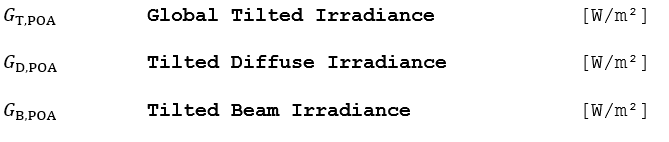# Perez Algorithm (Diffuse Sky)

1.) Find the factors that account for the respective angles of incidence of circumsolar radiation on the tilted and horizontal surfaces. The circumsolar radiation is considered to be from a point source.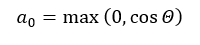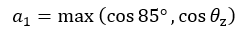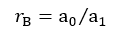2.) Compute the sky clearness as a function of the diffuse irradiance, direct normal irradiance, and zenith angle: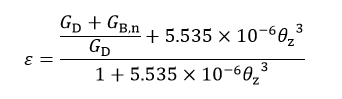3.) Find the brightness coefficients F1 and F2 from the lookup table in Figure 8 and the following equations: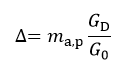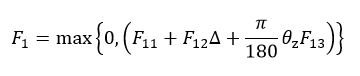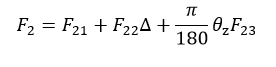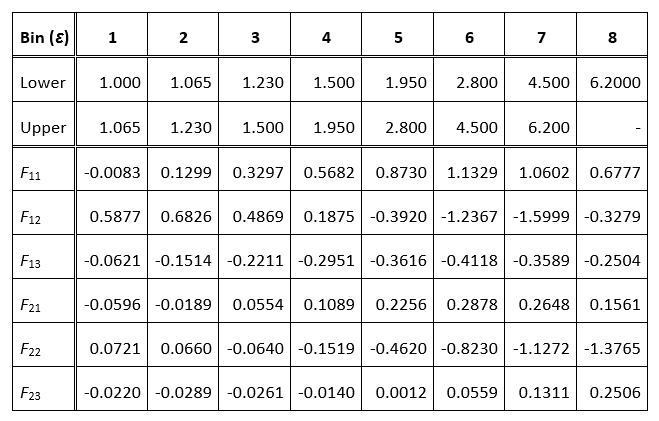### Figure 8. Coefficients of Slope Irradiance

4.) Compute the diffuse sky irradiance on the tilted plane: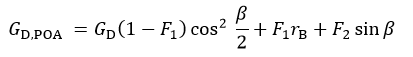# Hay Davies Algorithm (Diffuse Sky)

Compute the diffuse sky component on the plane of array using the direct beam transmittance from the DISC model: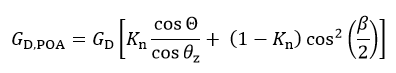# Common Algorithm (Beam and Diffuse Ground Components)

1.) Compute the beam component on the tilted plane as a function of the incidence angle and the tilt angle. Due to symmetry, incidence angles greater than 90° will have the same effect as those less than 90°. To avoid negative numbers, the absolute value is applied.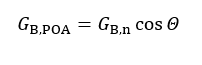2.) Compute the surface reflected irradiance on the tilted plane as a function of albedo and tilt angle.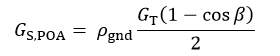3.) Sum the component irradiance to get the total irradiance on the tilted plane.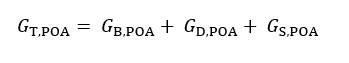## Reference

Muneer, T. Solar Radiation and Daylight Models, Elsevier, 2004, pp 102, 155, 156.

R. Perez, P.Ineichen, R. Seals, J. Michalsky, R. Stewart. Modeling Daylight Availability and Irradiance Component from Direct and Global Irradiance. Solar Energy 44, no 5, pp 271-289, 1990.

Hay, J.E., Davies, J.A., Calculations of the solar radiation incident on an inclined surface. Proc. of First Canadian Solar Radiation Data Workshop, 1980, 59. Ministry of Supply and Services, Canada.

# GTI DIRINT

This function derives direct normal irradiance (GB,n) and diffuse horizontal irradiance (GD) from the global tilted irradiance (GTi) using modifications to the DIRINT decomposition model and Perez transposition model. This allows for GTi to be input into an energy prediction, largely avoiding decomposition and transposition model errors (with the exception of calculations that require the direct/diffuse components, i.e. shading and IAM).

## Inputs## Outputs## Algorithm

This model implementation calculates a solution for incidence angles (θ) over and under 90° separately to allow solutions at times when the GTi measurement does not include any component of GD.

### 1. GTI DIRINT model for θ < 90°

Note that this model uses a modified (tilted) clearness index as described in the Clearness Index section. Unmodified DIRINT equations (see DIRINT Model) are used to calculate GB,n. GD can then be calculated using:Known errors are introduced when using the GTi as an input into the GTI DIRINT model (i.e. differences in proportions of diffuse and direct irradiance between global horizontal irradiance and GTi and the fact that GTi also includes ground reflected radiation). So, after Gand GB,n are calculated from the measured GTi using the reverse decomposition of GTI DIRINT, the forward transposition using Perez is used to calculate GTi as a check. The measured GTi and calculated GTi can then be compared and used as the iteration criteria in an optimization loop.

In the optimization loop, the initial calculation uses the measured GTi to calculate Kt. Subsequent iterations adjust the GTi until the Kt provides a GB,n and Gvalue such that a modeled GTi matches the measured GTi. The GTi value is adjusted using:Where i is the index corresponding to the iteration number, D is the difference between the modeled and measured GTi, and C is a coefficient to ensure an iterative solution (1.0 for i <3, 0.5 for 3 ≤ i < 10, 0.25 for 10 ≤ i < 20, and 0.125 for 20 ≤ i < 30). Often, only two iterations are needed. The following illustrates the flow chart for the model when θ < 90°.

##2. GTI DIRINT model for θ ≥ 90°

After GB,n and Gare calculated for θ < 90°, average early morning and late afternoon Kt‘ values are determined by averaging Kt‘ values for morning and afternoon instances when θ is between 65° and 85°. Besides Kt‘, the solution for GB,n requires Kt which is rearranged from the original DIRINT equation to solve for Kt instead of Kt‘: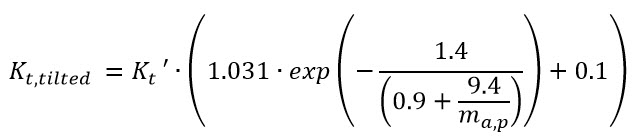The Gcan then be determined using:## Reference

Marion, B., A model for deriving the direct normal and diffuse horizontal irradiance from the global tilted irradiance.  Solar Energy 122 (2015), pp. 1037-1046.